# Solution assignment 01 Fractional functions and graphs

### Assignment 1

Given the function: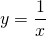Find:
the vertical asymptote;
the horizontal asymptote;
the intersection point with the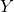-as if it exists;
the intersection point with the-as if it exists.

Based on these results sketch the result in the figure.

### Solution

This is the easiest fractional function. The vertical asymptote is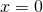. We find the horizontal asymptote by investigating the line to which the graph approaches whenor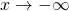and that is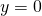. From this results we may conclude that there are no intersection points with either the- or the-axis

The graph is depicted in the following figure.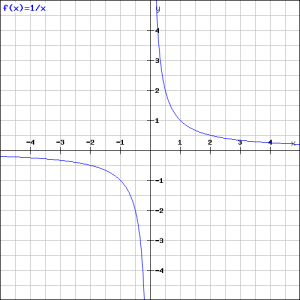0